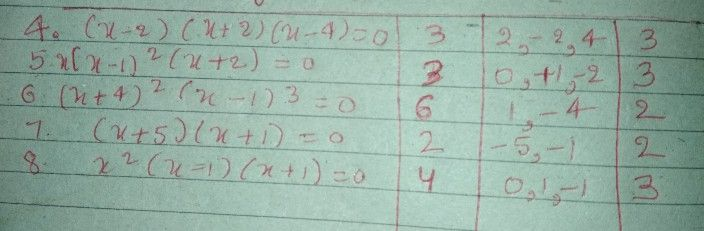Symbol
ProblemLearning Task $1$ Complete the table below by identifying the degree and real roots of polynomial equations (if a root occurs twice then use multiplicity 2 or if it occurs thrice use multiplicity 3) Three problems are done for you as example. Polynomial Equations Degree Roots Number of Real Roots $1$ $\left(x-1\right)\left(x+2\right)=0$ 2. $1.2$ $2$ $2$ $2x\left(x-3\right)\left(x+2\right)=0$ $3$ $\bar{2,0,3}$ 3\ $3$ $\left(x+2\right)^{2}\left(x-2\right)=0$ 4. $-2$ multiplicity $3,2$ $\left(x-2\right)\left(x+2\right)\left(x-4=0$ $4.$ $5.$ $x\left(x-1\right)^{2}\left(x+2\right)=0$ $\left(x+4\right)^{2}\left(x-1\right)^{3}=0$ $6.$ $\left(x+5\right)\left(x+1\right)=0$ $7$ $x^{2}\left(x-1\right)\left(x+1\right)=0$ $8.$
10th-13th grade
Algebra
Search count: 106
SolutionQanda teacher - Redox